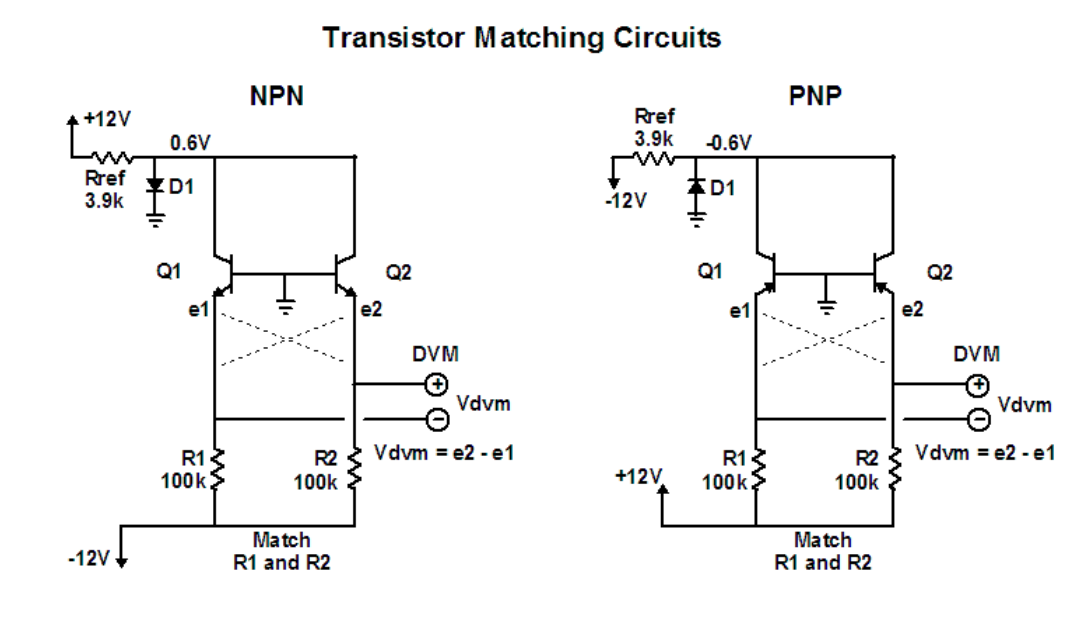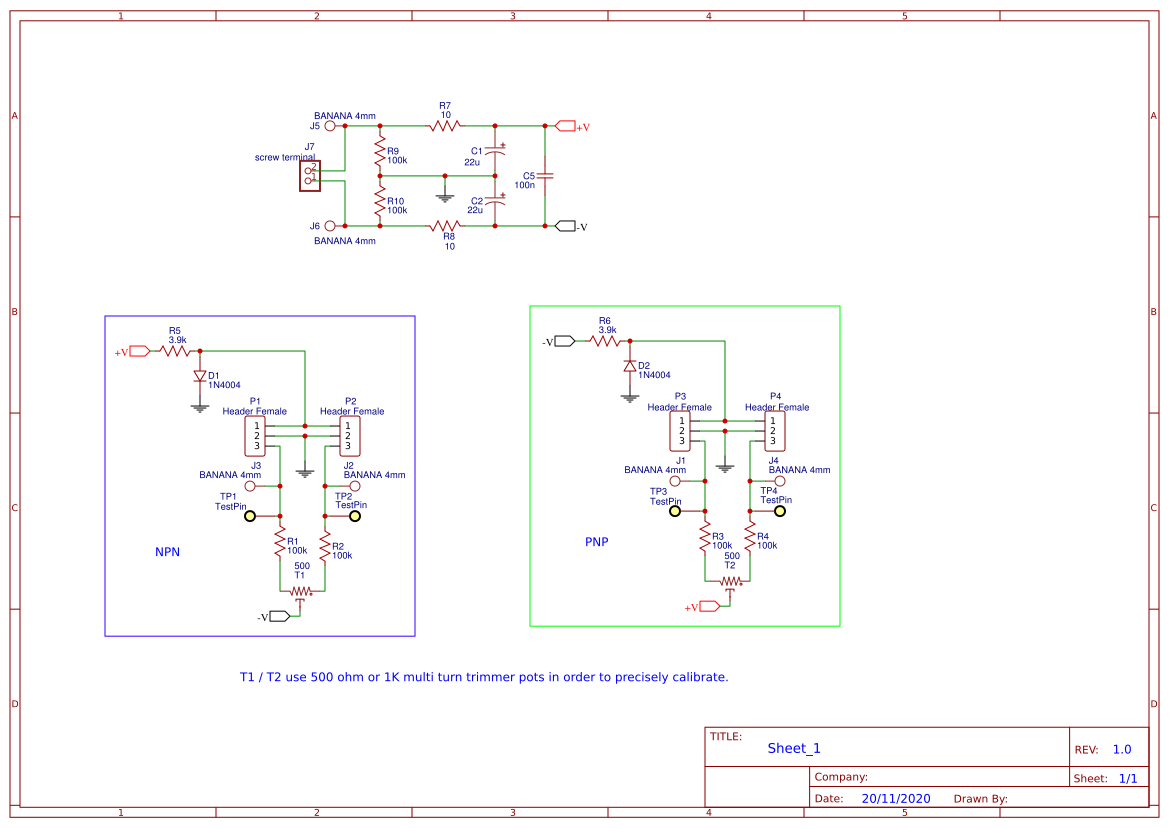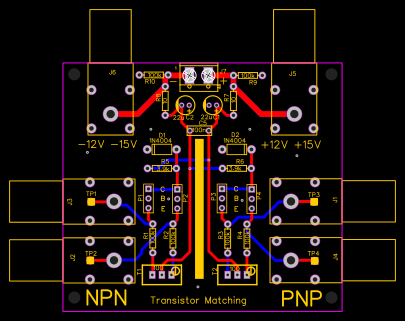Recommend Projects

# Transistor Matching Circuit

1 month ago 108
• Description
• Documents
• BOM
• Attachments
• Members

## Description

WARNING for the moment there is a problem, I still have not enough time to understand, the only one that I have in mind for the moment is that the NPN and PNP party can not function in parallel.

Transistor Matching Ian Fritz, October 2010

Purpose

• The purpose of this document is to describe a simple and accurate method of matching transistors for equal base-emitter voltage Vbe. Transistor matching is required in several situations. One is in selecting matched pairs for exponential or logarithmic circuits. Here it is actually the leakage currents that should be matched, but these are difficult to measure without special equipment, so Vbe is often used instead. A second use of transistor matching is for differential signal processing applications. These applications often have very stringent requirements on matching. For example, to make a high- performance operational transconductance amplifier with discrete components requires Vbe matching to within ~50 μV.

Ye Olde Way

• A common method of matching transistors is to use a fancy active current source to drive a stable current through the devices one at a time and to record the individual Vbe values. Then matched devices are selected based on the recorded values. There are a couple of limitations to this method. First, it is necessary to make highly precise measurements, since matching in the μV regime must be deduced from measured voltages near 0.6 V. Second, since the devices are characterized sequentially, there are possibly errors caused by temperature variations in the ambient surroundings.

Ye Easy Way

• Fortunately, there is a simpler, easier and more accurate method of Vbe matching. One such test test circuit is shown below. That's right, the circuit consists of just three resistors and a diode! Circuits for both NPN and PNP transistor types are given for completeness. The main idea behind this method is this: Since you want to know the difference between two transistors, why not set up a circuit to measure that difference directly, rather than having to make two separate measurements and then subtract the results?

Details, Details

• Let's look at the NPN circuit to see how this all works. Q1 and Q2 are the two transistors being compared. Rref and D1 set the voltage of the two collectors at 0.6 V. This is done merely to keep the transistors out of saturation, ie to have a finite voltage between the collector-base junction. ( Actually, the collectors can be biased to any positive voltage you want.) The two bases are connected together and to ground. Currents are drawn from the two emitters to the negative supply via matched resistors R1 and R2. The emitter voltages, e1 and e2, are connected to the DVM, which measures their difference. To start, imagine that we are in a fairy-tale land where Q1 and Q2 are perfectly matched and the resistors R1 and R2 are exactly equal. Then it's obviously that the circuit is perfectly symmetrical (electrically, that is) and so the DVM reads 0V. Now let's put one foot back into the real world and assume that the transistors are mismatched (but that the resistors are still equal). The DVM reads

Vdvm = e2 - e1 = (e2 - b) – (e1 - b) = Vbe2 - Vbe1 = dVbe,

where e1 and e2 are the emitter voltages, b is the base voltage, Vbe1 and Vbe2 are the base-emitter voltages and dVbe is the differential base-emitter voltage, which is the mismatch in Vbe between the two devices. Therefore, the DVM directly measures the transistors' mismatch voltage at the current level set by R1 and R2.More Details and a Trick

• Now all this is assuming that R1 and R2 are exactly equal. But any difference in these resistances contributes to the DVM reading. So now let's step back fully into the real world. It's important to match the resistors as closely as possible to make the erroneous contribution to the measurement small. But there will always be some difference between the resistors. Fortunately, the effect of this difference can be calculated and eliminated by a clever trick. Here's the clever trick: In addition to the measurement just discussed, a second measurement is made with the two transistors switched. This can easily be done by switching the emitter connections, as indicated crudely by the dashed lines in the diagram. (Switching the base and collector leads is not necessary, of course, because they are connected together.) When the second measurement is made, the contribution of the base-emitter voltages to the DVM voltage reverses sign. But the contribution from the resistor mismatch does not reversed sign. Thus dVbe is half the difference of the two readings, the subtraction eliminating the resistor error. Correspondingly, the resistor error, which we will call g, is given by half the sum of the two DVM readings. Once the value of g is known it can alway be subtracted from the first DVM reading to obtain the mismatch without needing the lead reversal.

Wha...?

• Didn't quite follow that? OK, lets go through it a bit more slowly. Call the two DVM readings Vdvm(a) and Vdvm(b). These are the DVM readings with the emitter leads as shown and then switched. The two readings are given by Vdvm(a) = Vbe2 – Vbe1 + g Vdvm(b) = Vbe1 – Vbe2 + g, so that (Vdvm(a) – Vdmv(b))/2 = (2Vbe2 –2Vbe1 + g – g)/2 = Vbe2 –Vbe1 = dVbe, (half the difference equals the mismatch), and (Vdvm(a) + Vdmv(b))/2 = (0 + 0 + g+ g)/2 = g, (half the sum is the error). Once g is known, then for future measurements the mismatch can be calculated as dVbe = Vdmv(a) – g, ie the transistor swap is no longer needed (but it doesn't hurt to do it anyway).

Bottom Line

• UlOK, OK, so what I actually do? It's really simple: (1) Find two accurately matched resistors of 100k or so. You can get close enough with a 4.5 digit DMM. Or if you have bux you can buy precision resistors. (2) Build the circuit, or even just set it up on a breadboard. Make it easy to reverse the emitter leads. Use appropriate sockets for your devices, so you can get them next to each other and at the same temperature. (3) Plug in your transistors, power up, and write down the DVM voltage. (Make sure you wait until the voltage is stable.) (4) Repeat (3) with the emitter leads swapped. (5) Calculate half the difference of the two readings. That's your mismatch. (6) Pick out the pairs that are good enough for your application. You are done!

Afterthoughts

• UlHere are some rambling miscellaneous thoughts.

What current?

• UlThe current through the transistors using R1 = R2 = 100k is about I = (12 - .6)V/100kOhm = 0.11 mA There is nothing special about this current level. You can change it easily by using a different resistor value. Or if you want to get really fancy, make the negative supply value variable. Then you can check matching at different current levels.

• UlInstead of finding super-matched resistors you can add a trimmer to the circuit and adjust the trimmer so that there is no error from resistor mismatch (g = 0). To modify the circuit here's what you do: (1) Disconnect the lower legs of the resistors from the power supply. (2) Connect a small value, multi-turn, cermet trimmer potentiometer across the two resistor leads. (3) Connect the slider of the trimmer to the power supply. (Where the resistors were originally connected.) (4) Measure the error g of the resistors as described above. (5) Adjust the trimmer and repeat (4) until you get g = 0.

Multiple devices

• UlThe above procedure is great if you are characterizing devices packaged in pairs. What do you do if you have a bunch of discrete devices and you want to find some good pairs? You do not have to use the above procedure for every possible pair! Here's what to do: (1) Plug any one of the transistors into the Q1 position -- and leave it there. (2) One at a time, plug the other devices into the Q2 position and measure the mismatch between them and Q1 using the procedure described above. (You do not need to swap the transistors with this method.) Write down all the mismatch numbers as you go along. (3) Understand that if two transistors have the same mismatch to Q1, then they are matched to each other. Based on this, your best pairs are those that have the most nearly identical numbers you wrote down. Hey, isn't this just the same as Ye Olde Way? No it is not. First, the measurements are still highly accurate direct differential measurements. Second, it doesn't matter if the ambient temperature changes some while you go through all your devices, because each device is at the same temperature as the reference transistor when the measurement is made. That's all that matters, but if you want to be sure you can (4) Measure the offset of the selected pairs directly, as in the first procedure.

An example

• UlI bought a bunch of CA3083 NPN arrays. Using Ye Olde Way I saw that the mismatch in these arrays was way less than the specified 1 mV. So I went through them all using the method above. I found that in eight of 20 chips, Q1 and Q2 were matched to better than 50 μV. Now that's nice to know, since they were pretty inexpensive. I was able to use them successfully as exponential converters in a number of VCO designs. B-b-b-but I have to match PNP transistors The procedure is exactly the same, except you use the circuit on the right.

Wheatstone?

• UlAnyone familiar with laboratory measurements will see the resemblance of the circuit to a Wheatstone bridge. Of course Wheatstone never heard of a transistor. But yes, the circuit works on the same principle, being a four legged arrangement with high sensitivity due to operating near a null condition.

If using this in combination with a volt meter please watch This video first as it tells you how to calibrate it first berfore use.

## Sheet_1 copy## PCB 1.0 copy## BOM

ID Name Designator Footprint Quantity BOM_Manufacturer Part
1 500 T1,T2 TRIMMER_DIPLOHM_P94 2 ?
2 TestPin TP1,TP2,TP3,TP4 TESTPIN 4 ?
3 22u C1,C2 CAP-D5.0XF2.0 2 ?
4 100n C5 CAP-SC_6.5X2.5XP5.0 1 ?
5 BANANA 4mm J1,J2,J3,J4,J5,J6 4MM PANEL SOCKET S16N-PC BANANA 6 571-0500
6 screw terminal J7 TERMINAL_BLOCK_2P_5 1 ?
7 10 R7,R8 AXIAL-0.3 2 ?
8 100k R1,R2,R3,R4,R9,R10 AXIAL-0.3 6 ?
9 3.9k R5,R6 AXIAL-0.3 2 ?
11 1N4004 D1,D2 DO-41 2 1N4004

## Attachments

None
Success
The owner does not allow comments in this project now